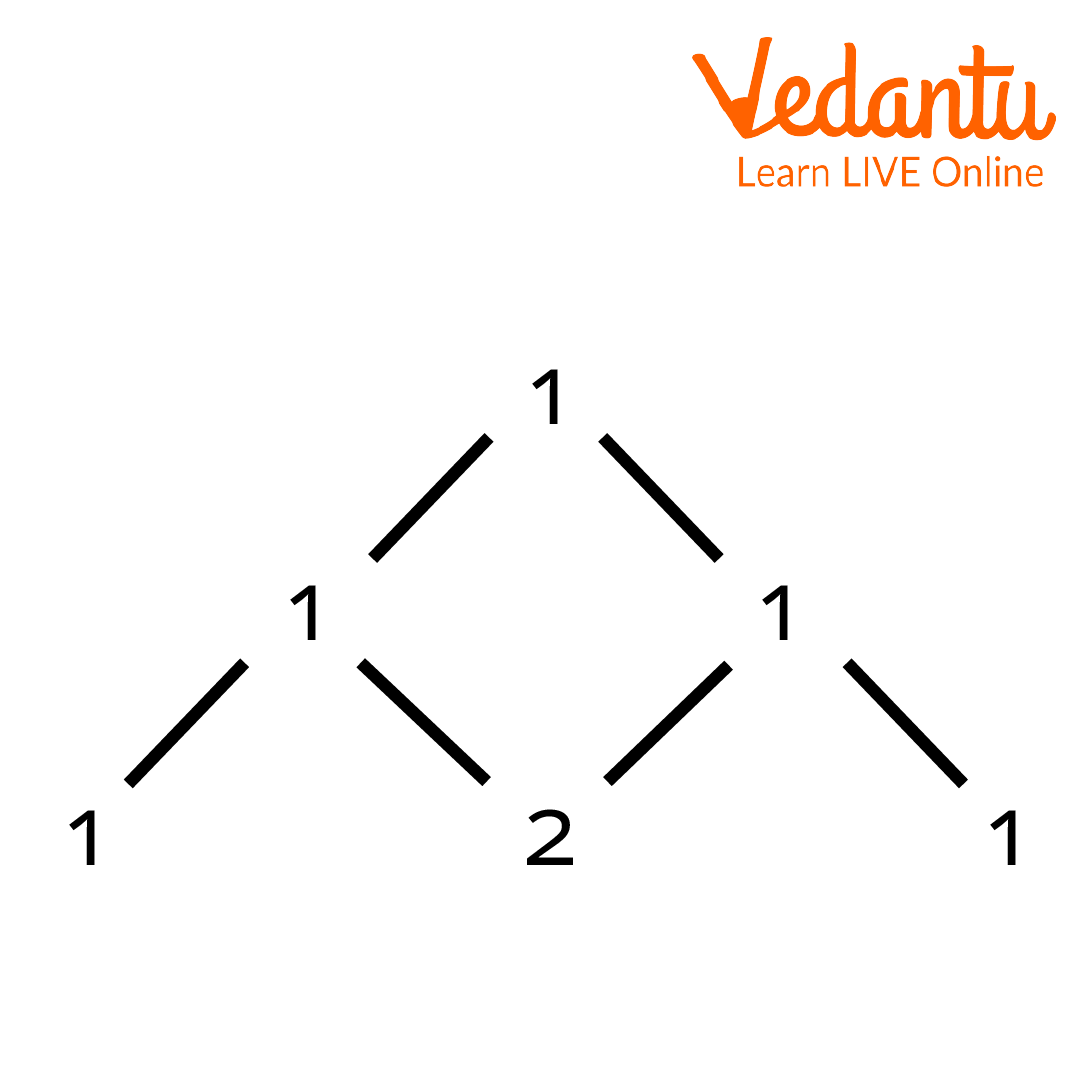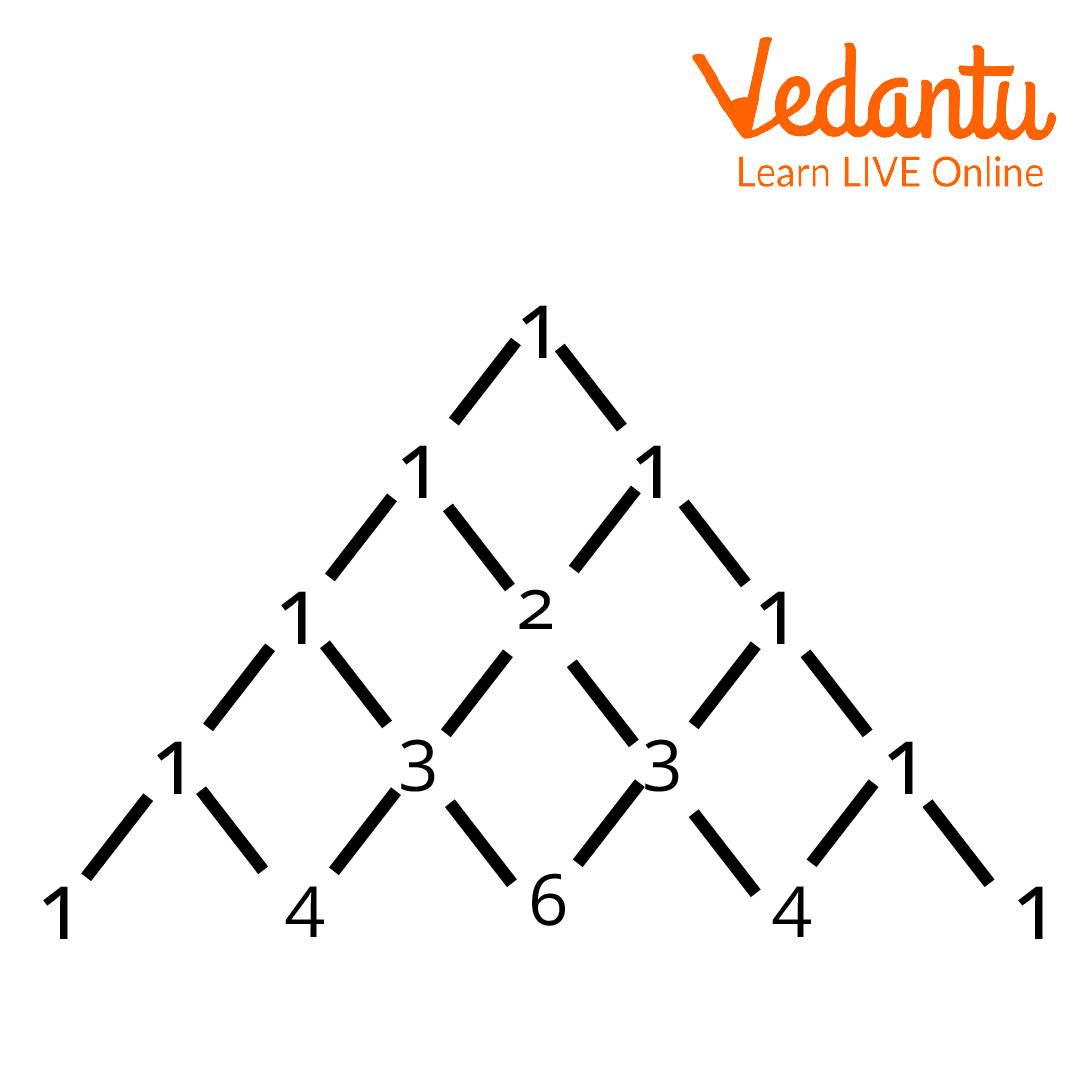Courses
Courses for Kids
Free study material
Free LIVE classes
More

# Pascal’s Triangle Binomial TheoremLIVE
Join Vedantu’s FREE Mastercalss

## An Overview of Pascal's Triangle Binomial Theorem

Pascal's triangle is a triangular array of binomial coefficients found in probability theory, combinatorics, and algebra. Pascal’s triangle binomial theorem helps us to calculate the expansion of ${{(a+b)}^{n}}$, which is very difficult to calculate otherwise. Pascal's Triangle is used in a variety of fields, including architecture, graphic design, banking, and mapping.

## History of Blaise PascalBlaise Pascal

Born: 19th June 1623

Died: 19th August 1662

Nationality: French

Contribution: He gave Pascal's Triangle Binomial Theorem to the world.

## Statement of the Pascal's Triangle Binomial Theorem

The Pascal’s Triangle Binomial Theorem states that the formula to find the entry of an element in the ${{n}^{th}}$ row and ${{k}^{th}}$ column of a Pascal’s Triangle is given by

$\left( \begin{array}{*{35}{l}}n \\k \\\end{array} \right)$

The formula listed below can be used to determine the components of the following rows and columns:

$\left( \begin{array}{*{35}{l}} n \\k \\\end{array} \right)=\left( \begin{array}{*{35}{l}} n-1 \\k-1 \\\end{array} \right)+\left( \begin{matrix}n-1 \\k \\\end{matrix} \right)$

## Proof of the Pascal's Triangle Binomial Theorem

Assuming$k\le n$,

$\left( \begin{matrix}n-1 \\k-1 \\\end{matrix} \right)+\left( \begin{matrix}n-1 \\k \\\end{matrix} \right)=\frac{(n-1)!}{(k-1)!(n-k)!}+\frac{(n-1)!}{k!(n-k-1)!}$

$=(n-1)!\left( \frac{k}{k!(n-k)!}+\frac{n-k}{k!(n-k)!} \right)$

$=(n-1)!\cdot \frac{n}{k!(n-k)!}$

$=\frac{n!}{k!(n-k)!}$

$=\left( \begin{array}{*{35}{l}} n \\k \\\end{array} \right)$

Hence proved.

## Application of the Pascal's Triangle Binomial Theorem

• We can write big binomial expansions with the help of Pascal’s triangle and we won’t have to use the formulas for binomial expansion.

• Concepts of Pascal’s Triangle are also used in so many Maths puzzles.

## Limitations of the Theorem

• For positive integral exponents, the theorem can’t be applied to commutative algebra.

• For negative or fractional exponents, the corresponding infinite series are subject to convergence criteria.

## Solved Examples

1. Expand and verify ${{\left( a+b \right)}^{2}}$.

Ans:Pascal’s Triangle

First, let’s write the generic equation without the coefficients:

${{(a+b)}^{2}}={{c}_{0}}{{a}^{2}}{{b}^{0}}+{{c}_{1}}{{a}^{1}}{{b}^{1}}+{{c}_{2}}{{a}^{0}}{{b}^{2}}$

The last row of the triangle gives us the value of coefficients:

${{c}_{0}}=1$, ${{c}_{1}}=2$, ${{c}_{2}}=1$

Now, let’s write the expansion with coefficients:

${{a}^{2}}+2ab+{{b}^{2}}$

2. Expand and verify ${{(a+b)}^{4}}$.

Ans:Pascal's Triangle (II)

First, let’s write the generic equation without the coefficients:

${{(a+b)}^{4}}={{c}_{0}}{{a}^{4}}{{b}^{0}}+{{c}_{1}}{{a}^{3}}{{b}^{1}}+{{c}_{2}}{{a}^{2}}{{b}^{2}}+{{c}_{3}}{{a}^{1}}{{b}^{3}}+{{c}_{4}}{{a}^{0}}{{b}^{4}}$

The last row of the triangle gives us the value of coefficients:

${{c}_{0}}=1,{{c}_{1}}=4,{{c}_{2}}=6,{{c}_{3}}=4\text{ }\!\!~\!\!\text{ and }\!\!~\!\!\text{ }{{c}_{4}}=1$

Now let’s write the expansion with coefficients:

${{(a+b)}^{4}}={{a}^{4}}{{b}^{0}}+4{{a}^{3}}{{b}^{1}}+6{{a}^{2}}{{b}^{2}}+4{{a}^{1}}{{b}^{3}}+{{a}^{0}}{{b}^{4}}$

3. Expand ${{(a+b)}^{5}}$.

Ans:Pascal’s Triangle III

First, let’s write the generic equation without the coefficients:

${{(a+b)}^{5}}={{c}_{0}}{{a}^{5}}{{b}^{0}}+{{c}_{1}}{{a}^{4}}{{b}^{1}}+{{c}_{2}}{{a}^{3}}{{b}^{2}}+{{c}_{3}}{{a}^{2}}{{b}^{3}}+{{c}_{4}}{{a}^{1}}{{b}^{4}}+{{c}_{5}}{{a}^{0}}{{b}^{5}}$

The last row of the triangle gives us the value of coefficients:

${{c}_{0}}=1,{{c}_{1}}=5,{{c}_{2}}=10,{{c}_{3}}=10,{{c}_{4}}=5\text{ }\!\!~\!\!\text{ and }\!\!~\!\!\text{ }{{c}_{5}}=1.$

Now let’s write the expansion with coefficients:

${{(a+b)}^{5}}={{a}^{5}}{{b}^{0}}+5{{a}^{4}}{{b}^{1}}+10{{a}^{3}}{{b}^{2}}+10{{a}^{2}}{{b}^{3}}+5{{a}^{1}}{{b}^{4}}+{{a}^{0}}{{b}^{5}}$

## Conclusion

Pascal's Triangle gives us the coefficients for an expanded binomial of the form ${{(a+b)}^{n}}$, where $n$ is the row of the triangle. The Binomial Theorem tells us we can use these coefficients to find the entire expanded binomial, with a couple of extra tricks thrown in.

## Important Points to Remember

• Each row's integers represent binomial coefficients.

• The figures on the second diagonal are composed of counting figures.

• The third diagonal numbers are triangular numbers.

• Tetrahedral numbers make up the fourth diagonal numbers.

• Pentatone numbers make up the fifth diagonal.

• The sum of the numbers on each row is a power of 2.

Last updated date: 26th Sep 2023
Total views: 103.8k
Views today: 3.03k

## FAQs on Pascal’s Triangle Binomial Theorem

1. What is a "Triangle"?

A triangle is a 2-dimensional closed shape with three sides, three angles, and three vertices. A triangle is a type of polygon. A few properties of the triangle are as follows:

• The sum of a triangle's three inner angles is always 180°.

• The total of any two triangle sides is always bigger than the length of the third side.

• A triangle's area is equal to half the product of its base and height.

Triangles can be categorised depending on their side lengths or angle measures. A few of them are Acute Triangle, Right Triangle, and Obtuse Triangle.

2. What is a fractal?

Fractal is a term used in Mathematics to describe geometric forms with detailed structures at arbitrarily small sizes, with a fractal dimension strictly exceeding the topological dimension. The pattern created by colouring only the odd numbers in Pascal's triangle closely resembles the fractal known as the Sierpinski triangle. When the number of rows increases, the likeness becomes increasingly precise; in the end, as the number of rows approaches infinity, the resulting pattern is the Sierpinski triangle, assuming a fixed perimeter.

3. What are polynomials?

A polynomial is an equation made up of variables, constants, and exponents that are mixed using mathematical operations including addition, subtraction, multiplication, and division. A polynomial can be categorised into 4 categories based on its degree. They are the cubic polynomial, the zero polynomial, the linear and the quadratic polynomial, and the biquadratic polynomial, where the highest power of each polynomial is zero, one, two, and four, respectively. The other main types of polynomials are trinomials, binomials, and monomials. The main reason for utilising Pascal’s triangle is to demonstrate how to expand binomials.